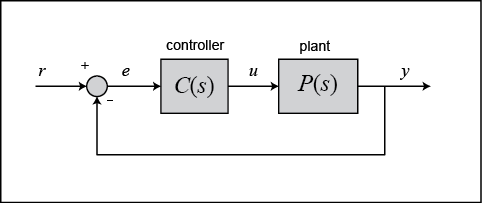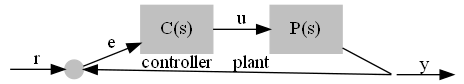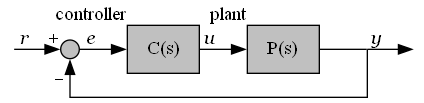# How to prevent Graphviz from moving nodes to accomodate an edge

I’m trying to reproduce the following figure using Graphviz:Here’s what I came up with so far:

``````digraph
{
rankdir="LR";
splines=polyline;

b [shape=circle, label="", style=filled, color=gray, width=0.2, height=0.2];
c [shape=box, label="C(s)", style=filled, color=gray, xlabel=controller];
d [shape=box, label="P(s)", style=filled, color=gray, xlabel=plant];
a [shape=point, color=transparent, label="a"];
e [shape=point, color=transparent, label="e"];
f [shape=point, color=transparent, label="f"];

c -> d [label="u"]
b -> c [label="e"]
a -> b [label="r"]
d -> e [dir=none]
e -> f [label="y"]
e -> b
}

``````

This gets me close but not quite there yet, as `dot` seems to move the nodes to accomodate the edge from `e` to `b` and not the other way round:How do I get it so that the edge from `e` to `b` bends around the nodes rather than displacing them?

I think you might be better off trying to use an SVG editor than Graphviz here; Graphviz is really good for when you have a graph and you need a computer to tell you how it should look. If you know how it should look and want the computer to replicate that look, you’re probably better off with the higher control given by SVG.

1 Like

It would help if you explained the isses with the two answers you got from stackoverflow.

You got pretty close. Here is what I came up with.With the following source

``````strict digraph "main"
{
layout="dot";
splines="ortho";
rankdir="TB";

{rank="same"; "b"; "c"; "d"; "a"; "e"; "f";}
"b"[ shape="circle" style="filled" fillcolor="gray" width="0.2" height="0.2" label="" ];
"c"[ shape="box" fillcolor="Gray"  style="filled" label="C(s)" xlabel="controller" ];
"d"[ shape="box" fillcolor="Gray"   style="filled" label="P(s)" xlabel="plant" ];
"a"[ shape="point" color="transparent" label="a" ];
"e"[ shape="point"  height="0" width="0" fixedsize="true" label="e" ];
"f"[ shape="point" color="transparent" label="f" ];
"c" -> "d"[ fontname="italic" label="u" ];
"b" -> "c"[ fontname="italic" label="e" ];
"a" -> "b"[ fontname="italic" labelfontname="Courier" label="r" headlabel="+" ];
"d" -> "e"[ dir="none" ];
"e" -> "f"[ fontname="italic" label="y" ];
"e" -> "b"[ labeldistance=1.75 labelfontname="Courier" headlabel="-" ];
}
``````

The main changes are:

1. Changed the direction from `rankdir="LR"` to `rankdir="TB"`
2. Put all the nodes on the same rank using a subgraph.
3. Used `splines="ortho"` instead of `splines="polyline"`
4. Used `headlabel` attributes for the + and - beside the arrowheads, and `labelfontname=Courier"` to make them show up better
5. Used the `labeldistance` attribute to push the - head label away from the arrowhead.

It’s still not completely identical. If you want identical you would have to draw it. In that case I recommend diagrams.net (aka ‘DrawIO’) which is free, runs in a browser, and lets you save files locally.

Hope this helps

2 Likes

Interesting. If others agree, we should probably provide this advice in our website. We should probably have an article discussing this situation.

As mentioned, Graphviz was not intended for much fine-tuning. It would be nice if we could at least understand the attachment points in the annotated SVG that can be loaded by inkscape (and probably Illustrator) but someone would have to track down exactly what code should be emitted.

1 Like